ZhouYongyi's Personal Blog# 吴恩达《机器学习》课程学习笔记（九）—— 支持向量机SVM

## 一、优化目标（Optimization Objective）

• 如果y=1，那么​$$h_\theta(x) \approx 1$$​且​$$\Theta^Tx \gg 0$$
• 如果y=0，那么​$$h_\theta(x) \approx 0$$​且​$$\Theta^Tx \ll 0$$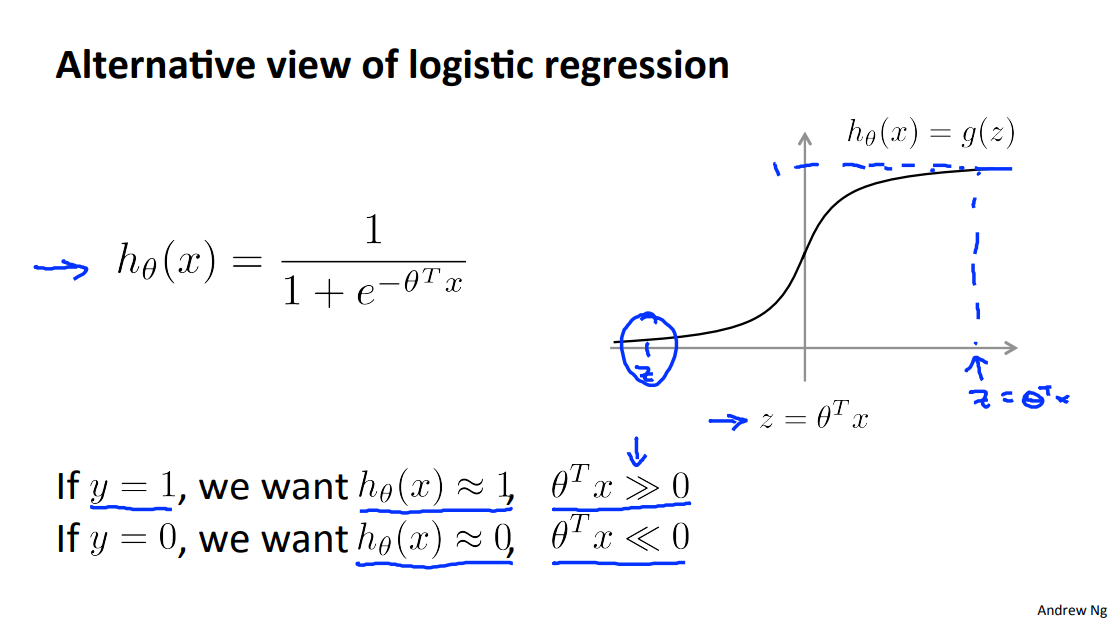\begin{align*}J(\theta) & = \frac{1}{m}\sum_{i=1}^m -y^{(i)} \log(h_\theta(x^{(i)})) – (1 – y^{(i)})\log(1 – h_\theta(x^{(i)}))\\ & = \frac{1}{m}\sum_{i=1}^m -y^{(i)} \log\Big(\dfrac{1}{1 + e^{-\theta^Tx^{(i)}}}\Big) – (1 – y^{(i)})\log\Big(1 – \dfrac{1}{1 + e^{-\theta^Tx^{(i)}}}\Big)\end{align*}

• 在y为0的时候，需要让$$θ^Tx$$比-1小，此时第一第二项都为零。
• 在y为1的时候，需要让$$θ^Tx$$比1大，此时第一项第二项都为零。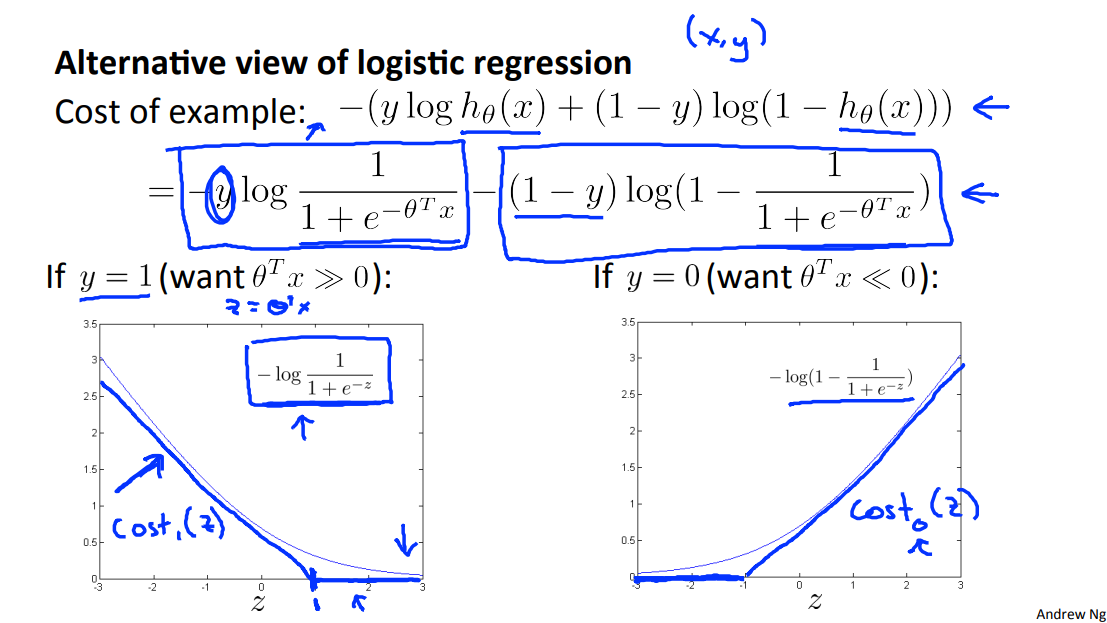$z = \theta^Tx$

$\text{cost}_0(z) = \max(0, k(1+z))$

$\text{cost}_1(z) = \max(0, k(1-z))$

$J(\theta) = \frac{1}{m} \sum_{i=1}^m y^{(i)}(-\log(h_\theta(x^{(i)}))) + (1 – y^{(i)})(-\log(1 – h_\theta(x^{(i)}))) + \dfrac{\lambda}{2m}\sum_{j=1}^n \Theta^2_j$

$J(\theta) = \frac{1}{m} \sum_{i=1}^m y^{(i)} \ \text{cost}_1(\theta^Tx^{(i)}) + (1 – y^{(i)}) \ \text{cost}_0(\theta^Tx^{(i)}) + \dfrac{\lambda}{2m}\sum_{j=1}^n \Theta^2_j \space\space\space\space\space\space(1.1)$

$J(\theta) = \frac{1}{\lambda}\sum_{i=1}^m y^{(i)} \ \text{cost}_1(\theta^Tx^{(i)}) + (1 – y^{(i)}) \ \text{cost}_0(\theta^Tx^{(i)}) + \dfrac{1}{2}\sum_{j=1}^n \Theta^2_j$

$J(\theta) = C\sum_{i=1}^m y^{(i)} \ \text{cost}_1(\theta^Tx^{(i)}) + (1 – y^{(i)}) \ \text{cost}_0(\theta^Tx^{(i)}) + \dfrac{1}{2}\sum_{j=1}^n \Theta^2_j \space\space\space\space\space\space(1.2)$

$h_\theta(x) =\begin{cases} 1 & \text{if} \ \Theta^Tx \geq 0 \\ 0 & \text{otherwise}\end{cases} \space\space\space\space\space\space(1.3)$

## 二、对大余量的直观理解（Large Margin Intuition）

• 如果想要预测y=1，那么我们需要使​$$\Theta^Tx \geq 1$$​（注意不仅仅是≥0）
• 如果想要预测y=0，那么我们需要使​$$\Theta^Tx \leq -1$$​（注意不仅仅是<0）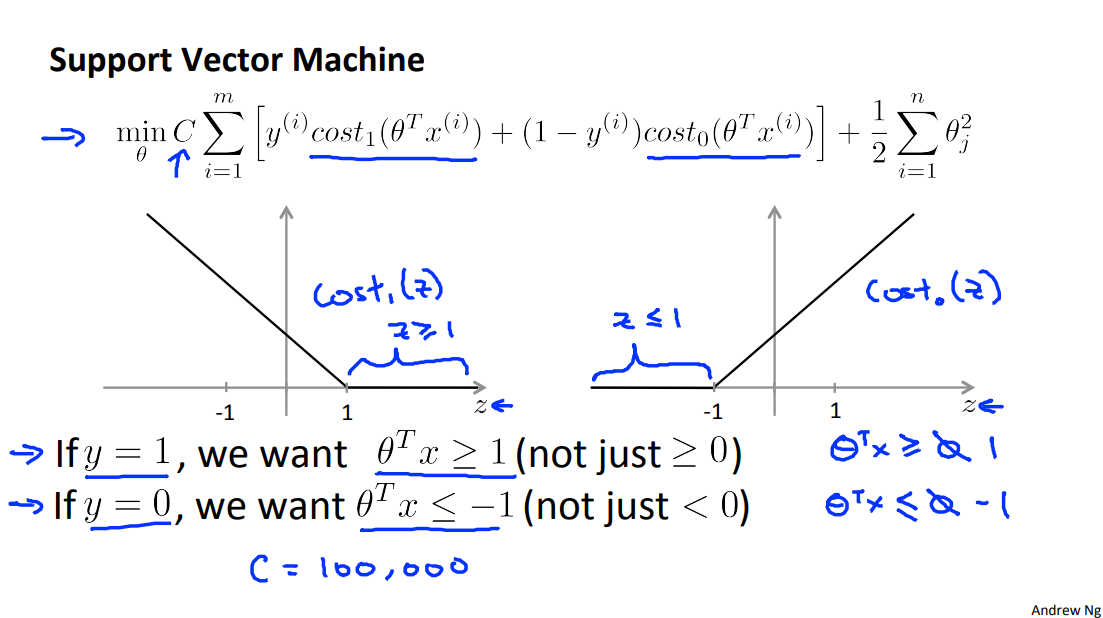\begin{align*} J(\theta) = C \cdot 0 + \dfrac{1}{2}\sum_{j=1}^n \Theta^2_j \newline = \dfrac{1}{2}\sum_{j=1}^n \Theta^2_j \end{align*}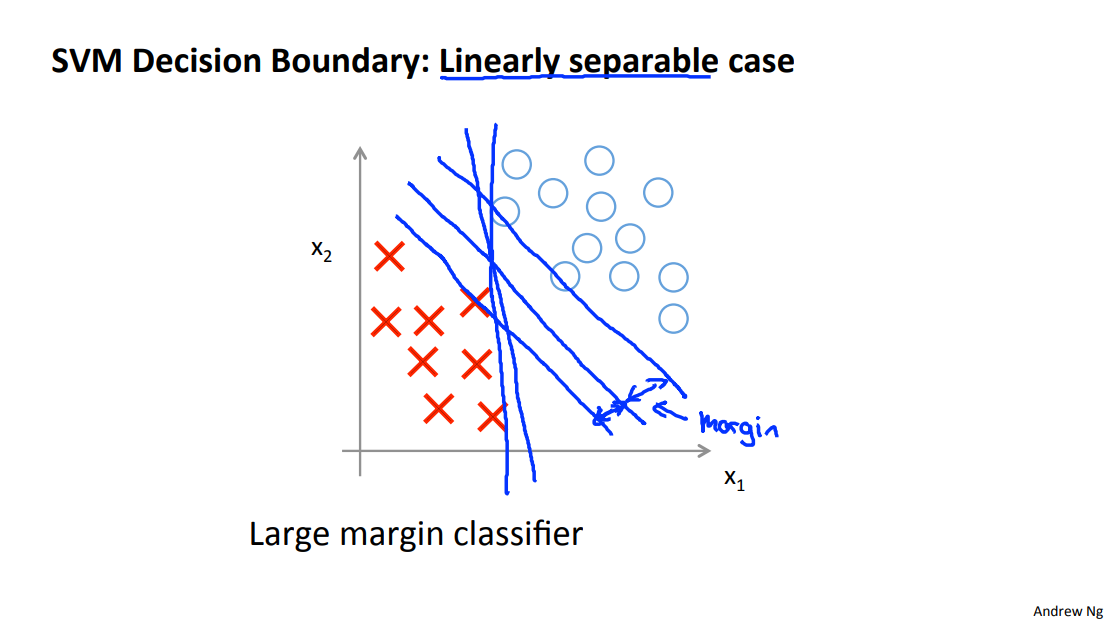• 样本与决策边界之间的距离称为余量（margin），由于SVM需要最大化余量，因而又被称为大余量分类器（Large Margin Classifier）
• SVM的大间距仅当C非常大的时候才能实现。
• 如果我们存在一些偏差较大的数据（outlier），又不想让其过分影响决策边界时，那么我们需要减小C的数值。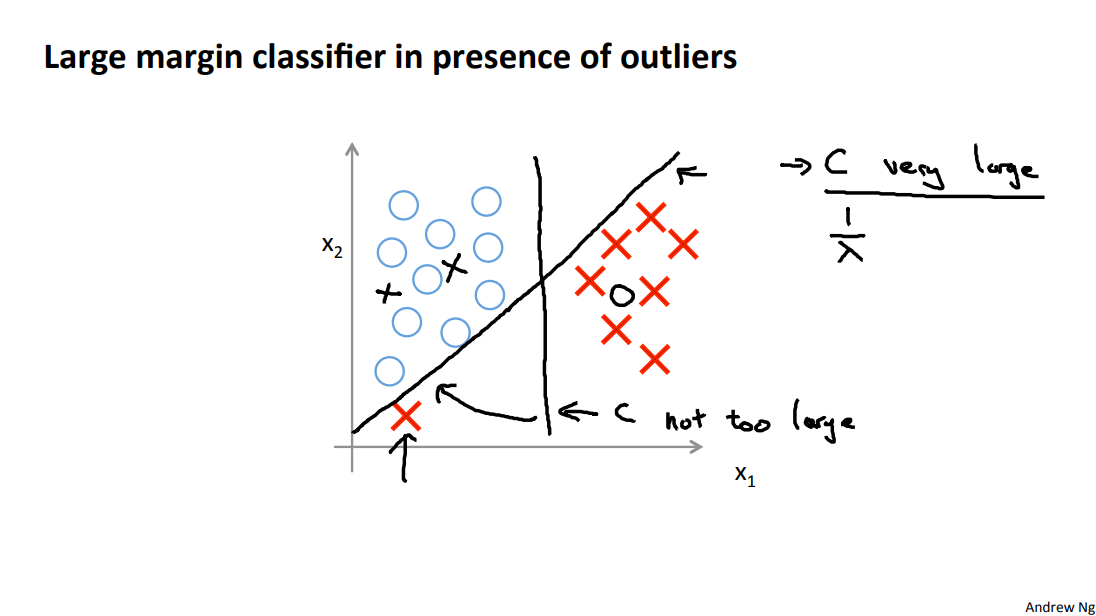## 三、大余量分类背后的数学原理（Mathematics Behind Large Margin Classification ）

### 1.向量内积（Vector Inner Product）

\begin{align*} u = \begin{bmatrix} u_1 \newline u_2 \end{bmatrix} && v = \begin{bmatrix} v_1 \newline v_2 \end{bmatrix} \end{align*}

$p = ||v|| \cos \theta$

$u^Tv= p \cdot ||u||$

$u^Tv = ||u|| \cdot ||v|| \cos \theta$

$u^Tv = v^Tu = p \cdot ||u|| = u_1v_1 + u_2v_2$

### 2.推广

\begin{align*}&\min_\Theta \dfrac{1}{2}\sum_{j=1}^n \Theta_j^2 \newline&= \dfrac{1}{2}(\Theta_1^2 + \Theta_2^2 + \dots + \Theta_n^2) \newline&= \dfrac{1}{2}(\sqrt{\Theta_1^2 + \Theta_2^2 + \dots + \Theta_n^2})^2 \newline&= \dfrac{1}{2}||\Theta ||^2 \newline\end{align*}

$\Theta^Tx^{(i)} = p^{(i)} \cdot ||\Theta || = \Theta_1x_1^{(i)} + \Theta_2x_2^{(i)} + \dots + \Theta_n x_n^{(i)}$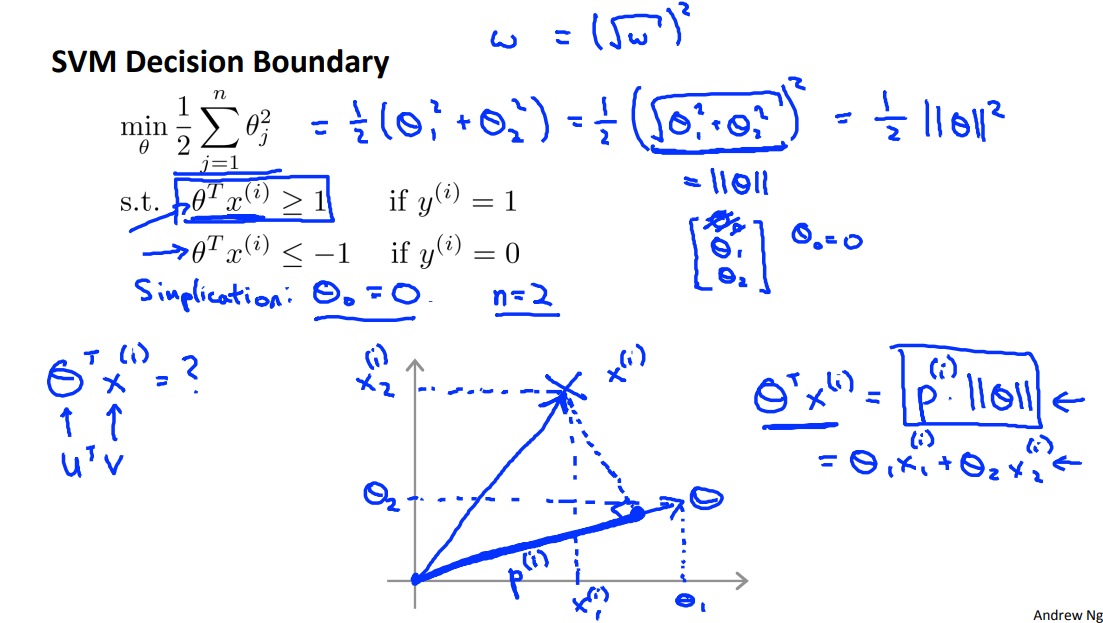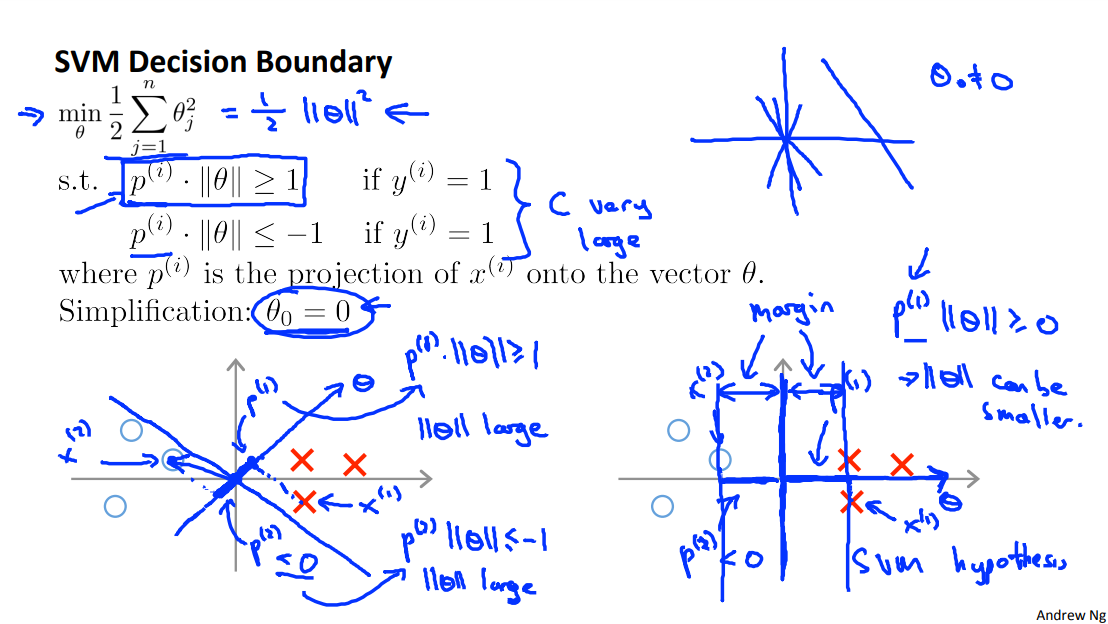## 四、核函数（Kernels）

### 1.Kernels I – 高斯核函数与“地标”

$h_\theta(x)=\theta_0+\theta_1x_1+\theta_2x_2^2+…$

$h_\theta(x)=\theta_0+\theta_1f_1+\theta_2f_2+… \space\space\space\space\space\space(4.1)$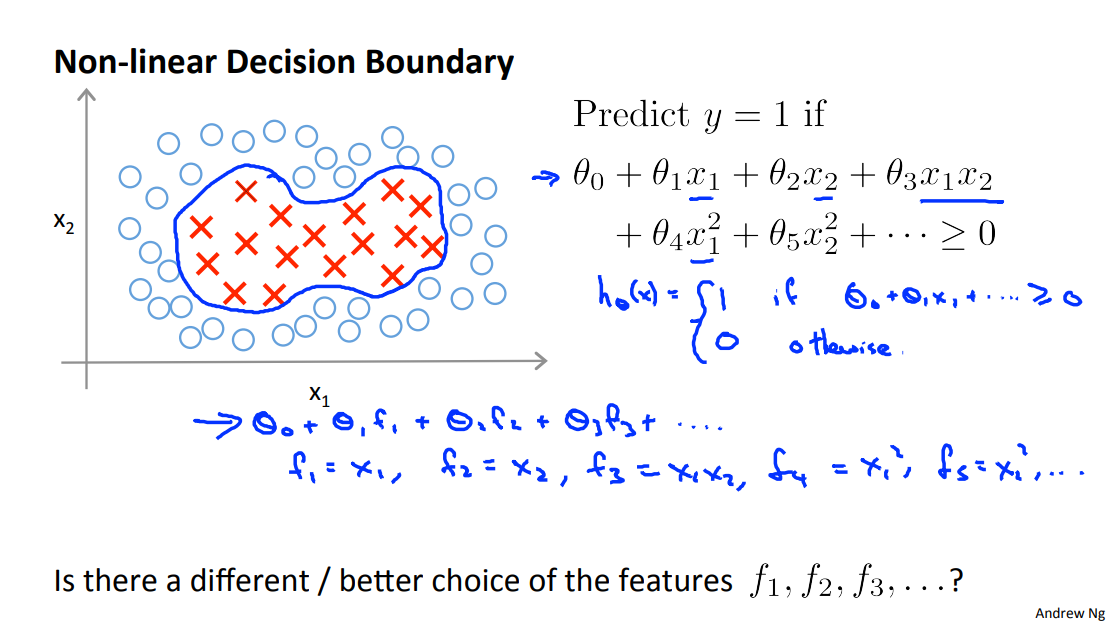$f_i = similarity(x, l^{(i)}) = \exp(-\dfrac{||x – l^{(i)}||^2}{2\sigma^2}) \space\space\space\space\space\space(4.2)$

$f_i = similarity(x, l^{(i)}) = \exp(-\dfrac{\sum^n_{j=1}(x_j-l_j^{(i)})^2}{2\sigma^2}) \space\space\space\space\space\space(4.3)$

• 如果​$$x \approx l^{(i)}$$​，即x离$$l^{(i)}$$​很近，那么​$$f_i = \exp(-\dfrac{\approx 0^2}{2\sigma^2}) \approx 1$$
• 如果x离​$$l^{(i)}$$​很远，那么​$$f_i = \exp(-\dfrac{(large\ number)^2}{2\sigma^2}) \approx 0$$

\begin{align*}l^{(1)} \rightarrow f_1 \newline l^{(2)} \rightarrow f_2 \newline l^{(3)} \rightarrow f_3 \newline\dots \newline h_\Theta(x) = \Theta_1f_1 + \Theta_2f_2 + \Theta_3f_3 + \dots\end{align*}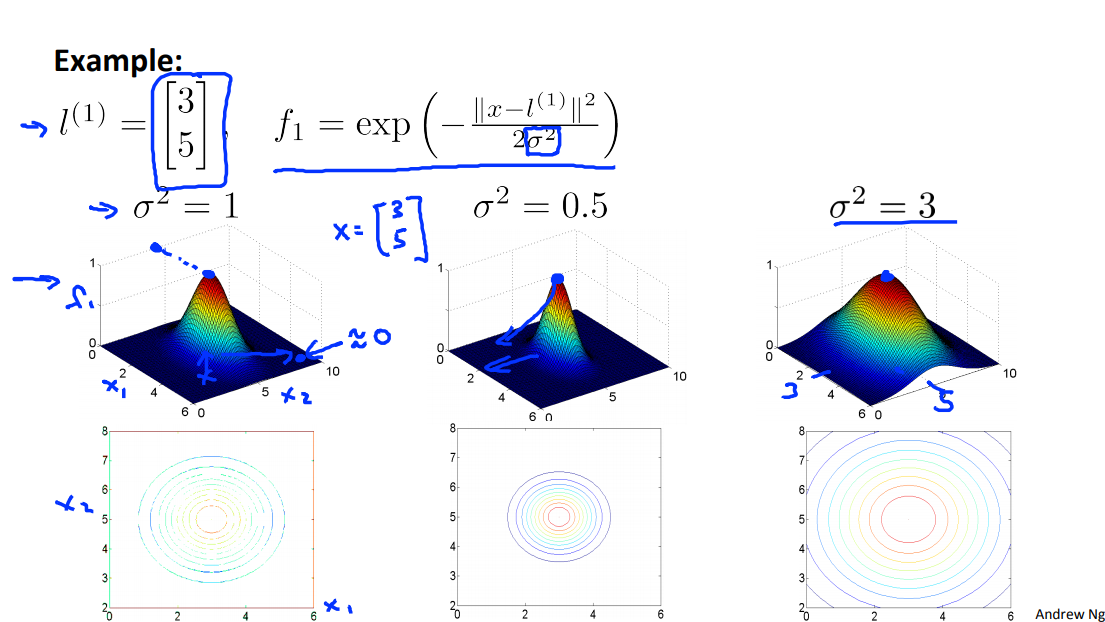### 2.Kernels II – 地标的选取

$f_1 = similarity(x,l^{(1)})，f_2 = similarity(x,l^{(2)})，f_3 = similarity(x,l^{(3)})$

$x^{(i)} \rightarrow \begin{bmatrix}f_1^{(i)} = similarity(x^{(i)}, l^{(1)}) \newline f_2^{(i)} = similarity(x^{(i)}, l^{(2)}) \newline\vdots \newline f_m^{(i)} = similarity(x^{(i)}, l^{(m)}) \newline\end{bmatrix}$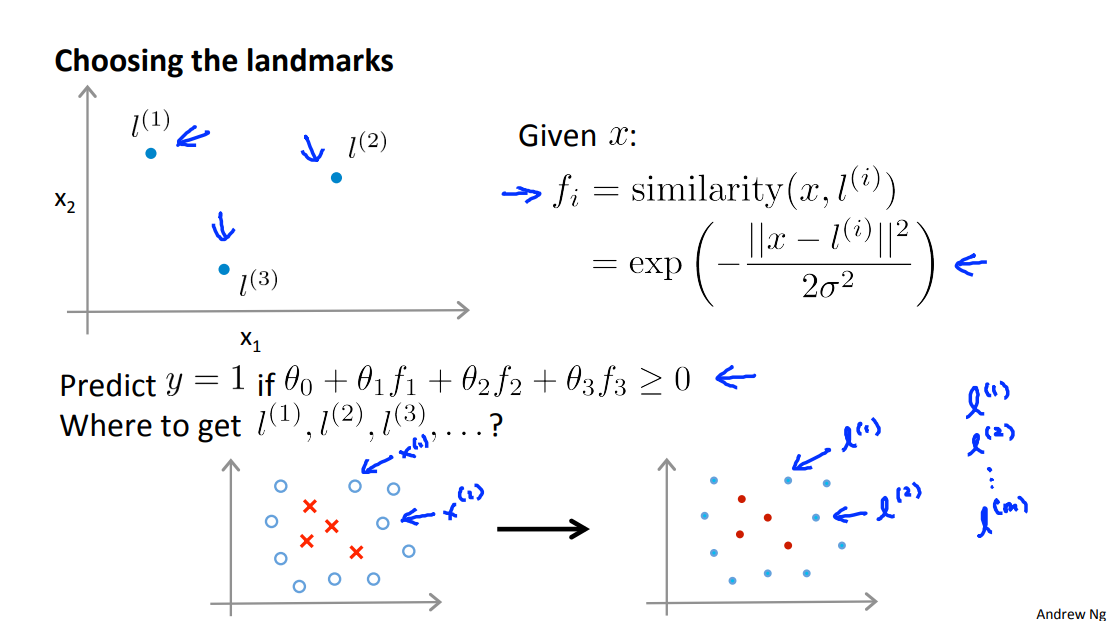$Cost = \min_{\Theta} C \sum_{i=1}^m y^{(i)}\text{cost}_1(\Theta^Tf^{(i)}) + (1 – y^{(i)})\text{cost}_0(\theta^Tf^{(i)}) + \dfrac{1}{2}\sum_{j=1}^n \Theta^2_j \space\space\space\space\space\space(4.4)$

### 3.选取SVM的参数（Choosing SVM Parameters）

• 如果C比较大，那么我们会有更高的方差、更低的偏差（过拟合）
• 如果C比较小，那么我们会有更高的偏差、更低的方差（欠拟合）

• 如果​$$σ^2$$​比较大，那么特征量变化会更加平滑，这意味着更高的偏差、更低的方差（欠拟合）
• 如果​$$σ^2$$​比较小，那么特征量变化会没那么平滑，这意味着更高的方差、更低的偏差（过拟合）

### 4.使用SVM算法（Using An SVM）

• 参数C的选择
• 核函数的选择
• 不使用核函数（其实是线性核函数）– 给出标准的线性分类器
• 决定什么时候n要大，什么时候m要小
• 高斯核函数需要选择​$$σ^2$$
• 决定什么时候n要小，什么时候m要大

### 6.逻辑回归与SVM算法的对比（Logistic Regression vs. SVMs）

• 如果n非常大（相对于m），那么使用逻辑回归，或者是无核函数的SVM算法（线性核函数）
• 如果n比较小，m适中，那么使用高斯核函数支持的SVM算法
• 如果n比较小，m非常大，那么手动地添加更多的特征量，然后使用逻辑回归或者不带核函数的SVM算法。

## 五、第六次编程作业

#### One Comment##### Wiki Yu

Good job! THX

$${}$$